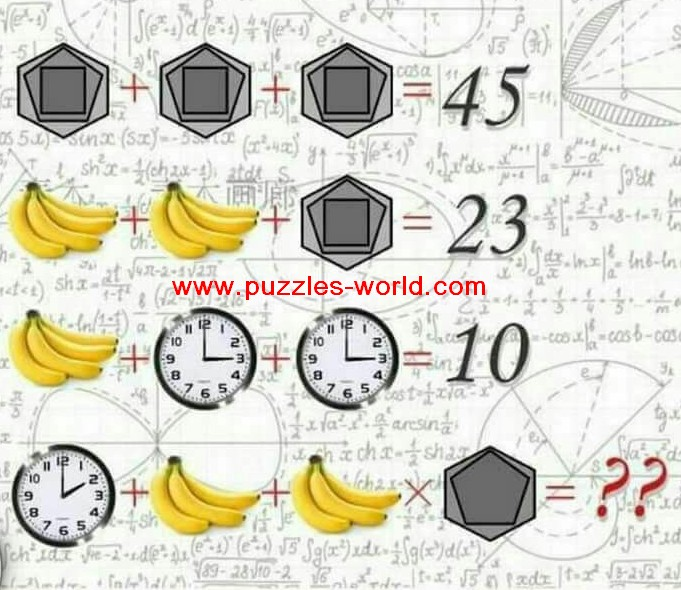# Clock Banana and Hexagon Logical Mathematics Puzzle### Clock Banana and Hexagon Logical Mathematics PuzzleHexagon + Hexagaon + Hexagon = 45
Bananas + Bananas + Hexagon = 23
Bananas + Clock + Clock =  10
Then,
Clock + Bananas + Bananas x Hexagon = ??

Think Logically and try to find the value !

Tips :
1. Look closely at the clock.
2. Number of Bananas.
3. Some thing regarding the sides of the Shapes figure.

Hint : Look Closely at the picture and try to logically relate the values for each item.

Logic :
From 1st the hexagon shape has value 15.
The shape has 15 edges(6 of hexagon,5 of Pentagon and 4 of Square)
From 2nd we get value of one bunch of bananas is 4.
So each banana has value of 1.
From 3rd we get that each clock has value of 3.
Which resembles the time on the clock which is 3.
Hence by using these insights, we get the last required values as
Clock = 2 (2 in the clock)
Bananas = 3 (3 bananas in the bunch)
Hexagon = 11 (Hexagon[6 sides] and pentagon[5 sides], so 6+5=11)
So required value is
2+3+3x11=?
2+3+33=? (Multiply first - Bodmas rule)
5+33=38 and hence the answer is 38.